How large is the classification error? What is the performance of the recognition  system? At the end this is the main question, in applications, in proposing novelties, in comparative studies. But how trustworthy is the number that is measured, how accurate is the error estimate?

The most common way to estimate the error of a classification system is by using a labeled test set and counting. There are a few, obvious, but often neglected conditions for obtaining a reliable, unbiased estimate of the error of the system when it is applied in practice. The test set should be representative for the future application. The best way to do this is to take a random sample of the objects that have to be recognized in the application and have them labeled by an expert.  This can be formulated as conditions on the test set:

• Test objects should be taken from the application and not collected in another way. Well known violations are the use of a different set of sensors or collecting the objects from a single site (e.g. a hospital) while the system will be used later on another set of sites.
• Test objects should be independently, randomly sampled in order to be representative. The removal of outliers, or attempts to make the set representative by selective sampling (e.g. a uniform distribution over subgroups) may harm this (e.g. as the subgroups have non-uniform probabilities).
• Labeling should be done by the best expert. It is not needed that this is the same one as used for labeling the training set, but usually it is, as the system will be better as the labels are better.

An additional and very frequently violated condition is:

• The test set should be used only once, and this result should be the result that is published. If the test set is used multiple times, e.g. in order to check whether changes in the system improve its performance, the test set is used implicitly for training. Assume that the changes make the system different, but do not improve its real performance, then after 10 changes the lowest of 10 random numbers with the same expectation (the true error) is reported, which is thereby positively biased.

### The worn out test set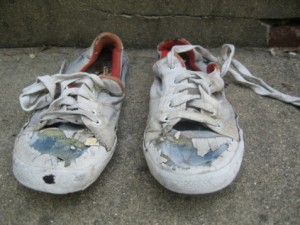In many practical applications it is almost impossible to satisfy the last condition as often a finite design set has been given by the customer. We will split it in parts for training and testing. The latter will be used during system design multiple times (or the split is performed multiple times, which is virtually the same). The result is that we get an optimistically biased idea about the performance of the system under construction. When the system is delivered and the customer makes a performance test himself by a test set that he has put aside for this purpose, we will be negatively surprised. The delivered system will perform worse than we estimated ourselves as we have used our test set many times in order to improve the system and the test set of our client is used just once. Our test set has been worn out by its use.

### Determining the error in the error

Suppose all conditions are fulfilled, including the single use of the test set. How accurate is the error estimate by counting the number of classification mistakes? Well, if the true error isthan the probability that a particular object is wrongly classified is.  The error estimated by the misclassified fraction of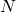test objects is:in which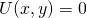for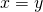and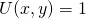if. The expectation of U isand its variance is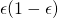. The expectation of the estimated error is, using the fact that the expectation of a sum is the sum over the expectations:which proves that the estimate is unbiased. The error in the estimate can be expressed in its standard deviation. First we compute the variance, which is the square of the standard deviation. We will make use of the fact that the variance of a sum is the sum of the variances if the terms are independent: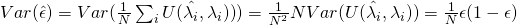Consequently the standard deviationof the error estimateis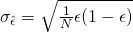It is nice to have an equation, but it is better to have a look at the real values. See the below table that lists the standard deviations for a various true errorsand sizes of the test set.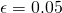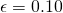0.031 0.044 0.069 0.095 0.126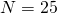0.020 0.028 0.044 0.060 0.080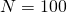0.010 0.014 0.022 0.030 0.040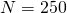0.006 0.009 0.014 0.019 0.025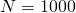0.0031 0.0044 0.0069 0.0095 0.01260.0020 0.0028 0.0044 0.0060 0.00800.0010 0.0014 0.0022 0.0030 0.0040

The standard deviation, however, is only a useful measure for the accuracy of the error estimate when its distribution is symmetric. This holds with a sufficient accuracy when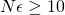. In that case the distribution ofis with good approximation Gaussian. Thereby an interval of two times the standard deviation to both sides has a probability of about 95%. The red figures in the table cannot be used as the condition for normality is not fulfilled. Moreover, a symmetric interval will often include negative numbers. For these small sample sizes more advanced techniques are needed. It is however clear that for these  error rates the given sample size are insufficient to find any reliable error estimate.

In conclusion, to avoid a large error in the error, the size of the test dataset should be at least in the hundreds and preferably in the thousands. For small class overlaps,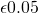this is even a must.

Filed under: ClassificationEvaluation